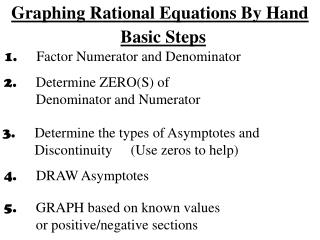DownloadDownload PresentationGraphing Rational Equations By Hand

# Graphing Rational Equations By Hand

Télécharger la présentation## Graphing Rational Equations By Hand

- - - - - - - - - - - - - - - - - - - - - - - - - - - E N D - - - - - - - - - - - - - - - - - - - - - - - - - - -
##### Presentation Transcript

1. Graphing Rational Equations By Hand Basic Steps 1. Factor Numerator and Denominator 2. Determine ZERO(S) of Denominator and Numerator 3. Determine the types of Asymptotes and Discontinuity (Use zeros to help) 4. DRAW Asymptotes 5. GRAPH based on known values or positive/negative sections

2. Special Behavior in Rational Equations #1: Vertical Asymptotes • x = a is a vertical asymptote if f(a) is undefined and a is a zero value of the denominator of f(x) only. • As x approaches a from the left or right side, f(x) approaches either ±∞ “Boundary you follow along” #1: x = a #2: x = a #3: x = a #4: x = a Examples: Zeros of Denominator that do not cancel Vertical Asymptotes at x = 1 and x = -6

3. Special Behavior in Rational Equations #2: Points of Discontinuity(Holes in Graph) • x = a is a point of discontinuity if f(a) is undefined • a is a zero value of the numerator and denominator of f(x). • Factor (x – a) can be reduced completely from f(x) #4: x = a #1: x = a #2: x = a #3: x = a Example:Zeros of Denominator that cancel Point of Discontinuity at x = -3 Vertical Asymptote at x = 5

4. Special Behavior in Rational Equations #3 Horizontal Asymptotes: • y = b is a horizontal if the end behavior of f(x) as x approaches positive or negative infinity is b. • Note: f(x) = b on a specific domain, but is predicted not approach farther left and farther right Case 1: Degree of denominator is LARGER thandegree of numerator Horizontal Asymptote:y = 0 (x – axis) Case 2: Degree of denominator is SAME AS degree of numerator Horizontal Asymptote:y = fraction of LEADING coefficients Case 3: Degree of denominator is SMALLER thandegree of numerator No Horizontal Asymptote: f(x) → ± ∞

5. Example 1:Identify the horizontal and vertical asymptotes, and any points of discontinuity. 0 0 [#1] Vertical: Horizontal: Discontinuity: [#2] Vertical: Horizontal: Discontinuity:

6. 0 Example 1:Continued 0 [#3] Vertical: Horizontal: Discontinuity: [#4] Vertical: Horizontal: Discontinuity:

7. Example 1:Continued 0 [#5] Vertical: Horizontal: Discontinuity:

8. Example 1:Continued 0 [#6] Vertical: Horizontal: Discontinuity:

9. Example 2:Sketch two possible graphs based on each description  Vertical: x = 2 Horizontal: y = - 3 Discontinuity: x = - 2  Vertical: x = -2, x = 1 Horizontal: y = 0 Discontinuity: x = 2

10. Example 2:continued  Vertical: x = -2 Horizontal: y = 3 Discontinuity: None  Vertical: x = 2, x = -1 Horizontal: y = - 2 Discontinuity: x = 0